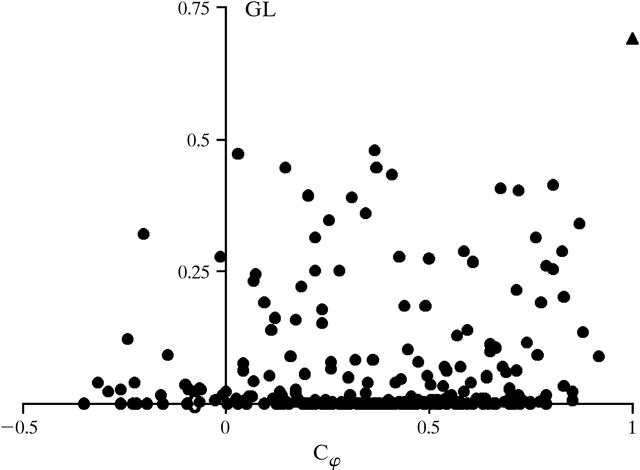disable zoom     view article Figure 2 GL-based phasing of the tRNAAsp–Asp RS complex using only the masks which have less than 0.12% of all points on the threefold and fourfold rotation axes. Cφ was calculated at d = 68 Å. GL was calculated at d = 60 Å. For the GL calculation, V = 0.3 of the cell, B = 8000, N = 100 atoms, ω = 0.86. The triangle corresponds to the variant closest to the correct solution.BIOLOGICALCRYSTALLOGRAPHY
ISSN: 1399-0047
Volume 56| Part 10| October 2000| Pages 1245-1252